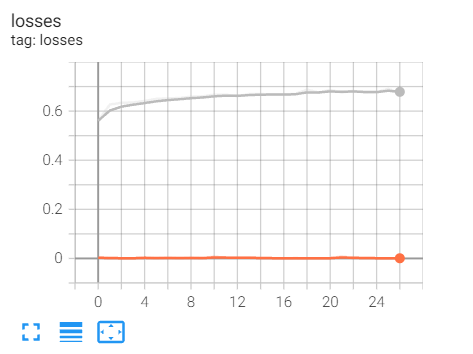# GRU overfit problems

Dear all,

I faced some problems with my overfitting model.grey refers to validation loss
orange refers to train loss.

batch_size = 16

My model as per below:
class SpeedClassificationPredictionModel(nn.Module):

``````def __init__(self, n_features, n_hidden=12, n_layers=2):

super().__init__()

self.n_hidden = n_hidden

self.gru = nn.GRU(

input_size=n_features,

hidden_size=n_hidden,

batch_first=True,

num_layers=n_layers,

dropout=0.8

)

self.regressor = nn.Linear(n_hidden, 1)   # output single number as prediction

def forward(self, x):

self.gru.flatten_parameters()    # sort out gpu memory during distributed training

_, hidden = self.gru(x)           # for gru

out = hidden[-1]                 # get the output from last layer

return self.regressor(out)
``````

loss function: MSE loss
First of all I will advise you remove the dropout as it is usually used for large networks and try using an Adam Optimizer `optimizer = torch.optim.Adam(net.parameters(), lr=0.001)`